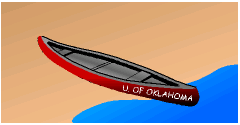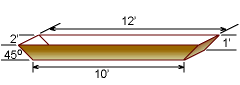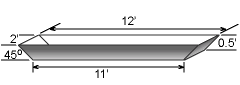Ch 2. Fluid Statics Multimedia Engineering Fluids PressureVariation PressureMeasurement HydrostaticForce(Plane) HydrostaticForce(Curved) Buoyancy
 Chapter 1. Basics 2. Fluid Statics 3. Kinematics 4. Laws (Integral) 5. Laws (Diff.) 6. Modeling/Similitude 7. Inviscid 8. Viscous 9. External Flow 10. Open-Channel Appendix Basic Math Units Basic Equations Water/Air Tables Sections Search eBooks Dynamics Fluids Math Mechanics Statics Thermodynamics Author(s): Chean Chin Ngo Kurt Gramoll ©Kurt GramollFLUID MECHANICS - CASE STUDY IntroductionCanoe In preparation for the upcoming Concrete Canoe Competition, the University of Oklahoma chapter of the American Society of Civil Engineers (ASCE) is currently soliciting design ideas from its student members. Two canoe design layouts (as shown in the figures) have been received so far. These designs are similar in shape but with different dimensions. Assume either canoe weighs 120 pounds and the total weight of the paddlers and gear is 630 pounds.Design Diagram ADesign Diagram B Question Based on a simple buoyancy analysis, determine if the designs will float or sink by calculating the submerged waterline. Approach For a simple analysis, use a single concentrated load instead of distributed load. Assume a water density of 1.94 slugs/ft3.

Practice Homework and Test problems now available in the 'Eng Fluids' mobile app
Includes over 250 problems with complete detailed solutions.
Available now at the Google Play Store and Apple App Store.